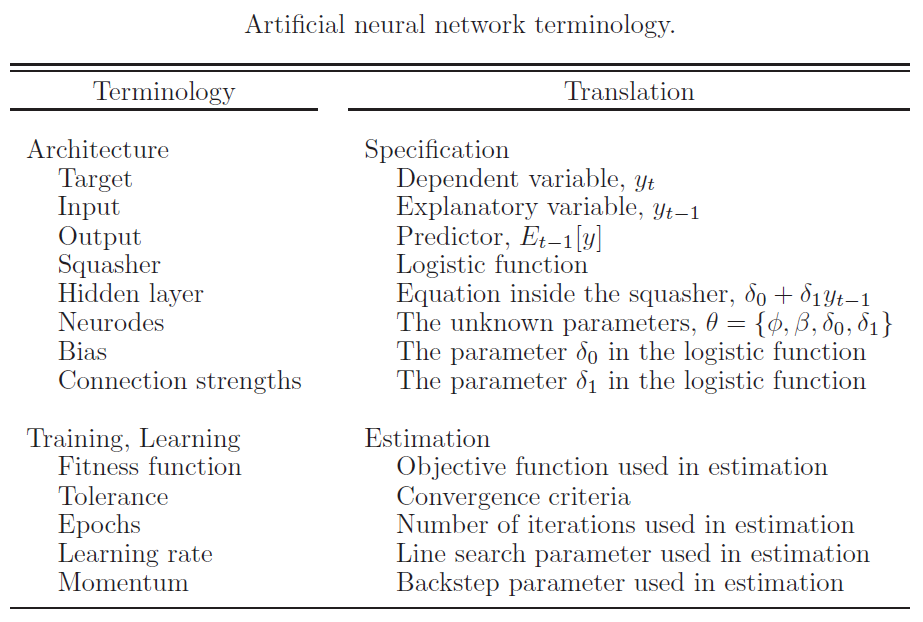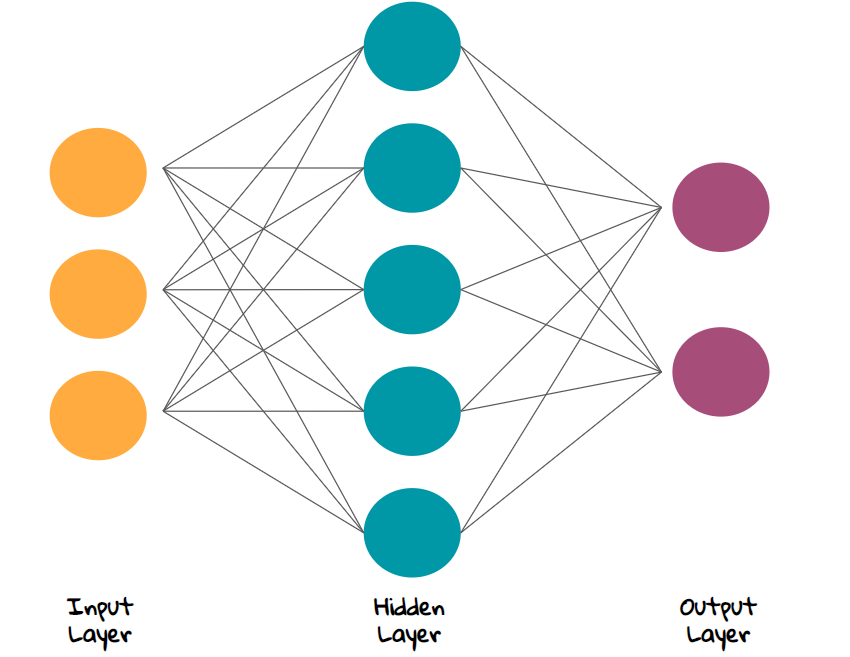@fanxy 2020-06-07T13:12:36.000000Z 字数 8593 阅读 1594

# 第十六讲 系统性金融风险III：大数据分析方法简介与应用

樊潇彦 复旦大学经济学院 金融数据

# 1. 为什么需要大数据方法？

• 以OLS估计为例，假定 $y = X\beta + \varepsilon$，在一定的条件下$\hat y = X\beta^{OLS}$，其中：
• $y\in \left\{1,2...K\right\}$ 为离散变量时，在一定的假定条件下，用Logit模型可以估计 $y=k$ 的概率：

# 2. 金融和商业预测中常用的大数据方法

## 2.2 岭回归(Ridge regression)与套索回归(LASSO regression)

• 岭回归估计量定义如下（损失函数 $V\left( \varepsilon \right) = \varepsilon^T\varepsilon$，调节参数 $\lambda\ge 0$）：
• 套索回归估计量定义如下（损失函数 $V\left( \varepsilon \right) = \varepsilon^T\varepsilon$，调节参数 $\lambda\ge 0$$\bf{1}$为单位向量）：

James et al.(2015)指出：“一般情况下当一小部分预测变量是真实有效的，而其他预测变量系数非常小或者等于零时，LASSO要更为出色；当响应变量是很多预测变量的函数，并且这些变量系数大致相等时，岭回归较为出色。”

## 2.3 基于树的回归方法(tree-based regression)

1. 回归树(regression tree)

2. 袋装法(bagging, or bootstrap averaging)

3. 随机森林(random forest)
Breiman(2001)提出的随机森林(random forest)方法也可以视为对回归树方法的一种改进，基本思想是每次在对树做分叉之前，先随机选出 $m$ 个解释变量，对剩下的变量做树的分叉，调节参数是树的深度和 $m$

4. 提升树(tree boosting)

1. 令迭代次数 $m=0$，使用原始数据 $d^0=(x_i,y_i)$ 生成树 $\hat{y}^{0}(d^0)$，保存预测 $f^0_i=\alpha \cdot \hat{y}^0_i$，其中
$\alpha \in [0,1]$ 是一个收缩调整参数。保存残差 $e_i^{0}= y_i - f^0_i$。更新 $m=1$
2. 在第 $m$ 次迭代中，使用数据 $d^m=(x_i,e_i^{m-1})$ 来生成树 $\hat{y}^{m}(d^m)$。保存预测 $f^m_i = f^{m-1}_i + \alpha \cdot \hat{y}^m$。保存
残差$e_i^{m}=y_i - f^m_i$。更新 $m=m+1$
3. 重复步骤2，直到 $m>M$

## 2.4 神经网络(neural network, NN)

Martin et al.(2013) 第19章给出了神经网络和AR(1)回归的关系：Shi(2020)指出，从统计学家的角度来看神经网络是一种特殊类型的非线性模型。$k$ 层神经网络模型可以写成：• $k$ 个隐藏层(hidden layer) 有 $J_{k-1}$ 个输入$a_j^{(k-1)}, j=1,2,...J_{k-1}$$a_j^{(0)}=x_j$ 为原始输入数据。
• $z_l^{(k)}$ 为第 $k$ 个隐藏层的输出，最后一层的输出为估计值 $\hat y_l = z_l^{(K)}$$z_l^{(k)}$通常采用线性形式，所有的 $w$ 为待估计参数。
• $k$ 层的输出经激活函数 $g(\cdot)$ 变换，成为下一层的输入。 激活函数可以是一个特征函数或一个简单的非线性函数，常用的选择有sigmoid函数 $1/(1+exp(-x))$ 或整流线性单元等。

# 3. 系统性风险分析的应用

## 3.1 Diebold and Yilmaz(2003)：风险溢出指数(SOI)

$E_t\left[\bf{y_{t+1}}\right]=\bf{y_{t+1,t}}=\mathbf{\Phi y_t}$，则对未来一期的预期误差(1-step-ahead error)等于：

# 4. 补充阅读材料

• Gu et al.(2020)调查了1957年至2016年交易的3万只个股，利用机器学习(ML)的几种技术研究了数百种可能的预测信号，结果发现在这项具有挑战性的任务中，ML比传统分析有显著优势。Chicago Booth Review总结了文章的几个主要发现:(1)决策树和神经网络是预测资产价格最有效的ML形式。使用决策树，计算机学会以扩张性流程图的方式进行思考，并进行多次迭代。神经网旨在模仿生物神经网络，这种技术在模拟非线性和互动模式方面特别有用。(2)在研究人员调查的近100个特征中，最成功的预测因素是价格趋势、流动性和波动性。(3)机器在预测大的、流动性强的股票的风险溢价方面比预测小的、流动性较差的股票要好。Matlab程序和数据可从Dacheng Xiu 的个人主页下载。

• Das, S.R. 2017: Data Science: Theories, Models, Algorithms, and Analytics, Online textbook

# 参考文献

1. Bajari, P., D. Nekipelov, S.P. Ryan, and M.Y. Yang 2015: Machine learning methods for demand estimation, American Economic Review, Vol.105(5), P481–85
2. Barigozzi, M. and C. Brownlees 2019: NETS: Network estimation for time series, Vol.34(3), P347-364， R package: nets
3. Diebold, F.X. and K. Yilmaz 2009: Measuring Financial Asset Return and Volatility Spillovers, with Application to Global Equity Markets, The Economic Journal, Vol.119(534), P158-171
4. Gu, S.H., B. Kelly, and D.C. Xiu 2020: Empirical asset pricing via Machine Learning, Review of Financial Studies, Vol.33(5), P2223-2273
5. James, G., D. Vitten, T. Hastie, R. Tibshirani 著：《统计学习导论：基于R应用》，王星译，机械工业出版社，2015，（在线课程链接
6. Klößner, S. and S. Wagner 2009: Exploring All Var Orderings For Calculating Spillovers? Yes, We Can! — A Note On Diebold And Yilmaz(2009), Journal of Applied Econometrics, Vol.29(1), P172-179, Data and Code
7. Lin, W., Z.T. Shi, Y.S. Wang, and T.H. Yan 2020, Unfolding Beijing in a Hedonic Way, Working Paper
8. Martin, V., A. Hurn, and D. Harris 2013: Econometric modelling with time series: specification, estimation and testing(Themes in Modern Econometrics), Cambridge University Press, United States of America
9. Shi, Z.T. 2020: Prediction-oriented algorithms, Lecture Notes in Computational Methods in Economics(Econ5170, CUHK)• 私有
• 公开
• 删除